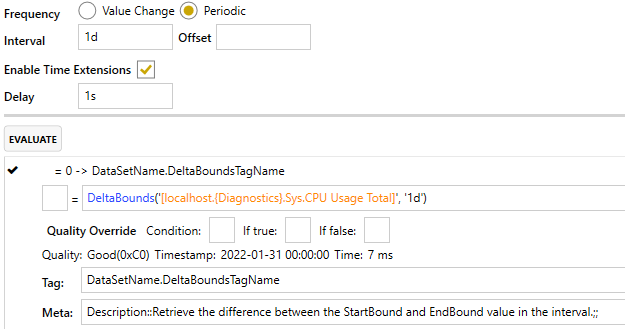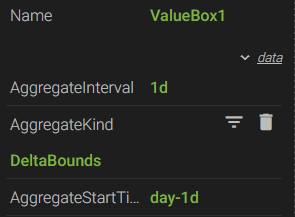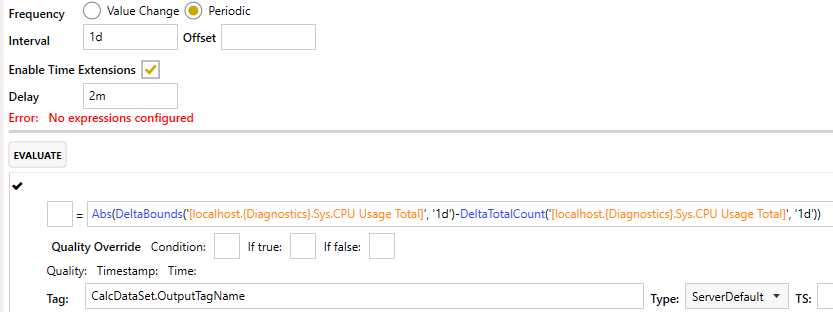0# Calc Server - Volume of Container

Hi, all.  I have data of a container that shows the amount of fluid in a contained (in gallons).  Some additional volume is pumped throughout the day and some is pumped out as well, and I was hoping there's an easy way of telling me how much I am processing in 24 hours without manually calculating it.

3replies Oldest first
• Oldest first
• Popular•Hi sagar

We do have a DeltaBounds aggregate that might suffice for what you are trying to do. Essentially, it finds the difference between the EndBound and StartBound value within the interval. In my screenshot, I have it set to calculate periodically once a day at midnight.If you don't need to historize the data, you could just use a ValueBox in Axiom using the DeltaBounds aggregate with an interval of "1d" and a startTime of "d-1d". That would give you yesterday's delta from midnight to midnight. You could shift the startTime if needed.Like
•Hi sagar

I think this calculation may be what you're looking for:As mentioned previously, the DeltaBounds finds the difference between the start and end of the interval. In addition to that, the DeltaTotalCount aggregate finds all of the increments (or additions) throughout the interval, ignoring the subtractions. So I'm finding the difference throughout the interval, then subtracting out the increments so that all you are left with is the "subtractions". I'm taking the absolute value of the entire expression so that it always returns a positive value.

A couple caveats...

1. If fluid is pumped in at the same rate fluid is pumped out, I'm not sure we would be aware of that because the amount would be the same.

2. I set the "Delay" field to 2 minutes. This is for the DeltaBounds portion of the calculation. In order to calculate the DeltaBounds value, we need to know the EndBound value of the interval. If the value doesn't change frequently the historian extends the last known value about once a minute. Building in a 2 minute delay will ensure we have an Endbound value to do the calculation.

Like
Like Follow